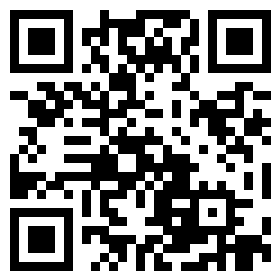# 实验吧：print and scan

```import numpy as np
from PIL import Image
f = open('Paint&Scan.txt','r')
line = line.replace('(','').replace(')','').split('\r\n')[:-1]
im = np.array([*280]*280)

for i in range(len(line)):
x = int(line[i].split(','))
y = int(line[i].split(','))
im[x][y]=0

narray = np.matrix(im,dtype='uint8')
img = Image.fromarray(narray)
img.convert('L')
img.show()
```[2;3Rer>